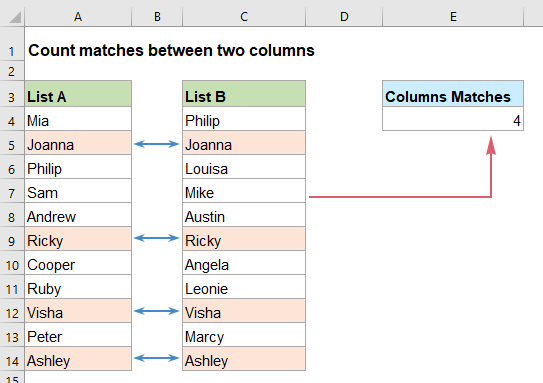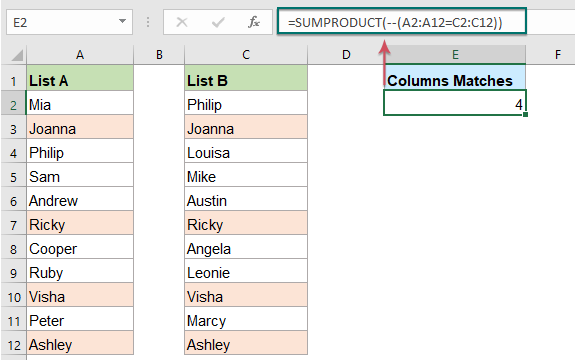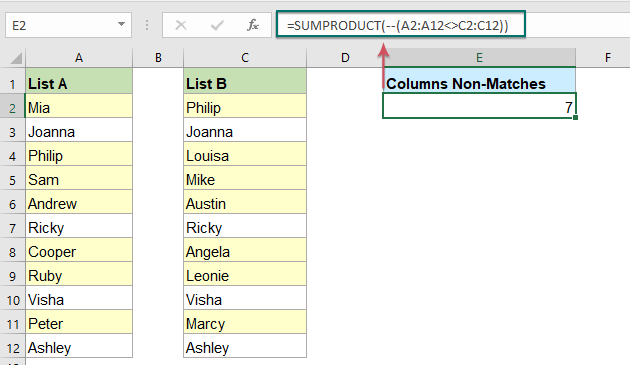## Count matches between two columns in Excel

For example, I have two lists of data in column A and column C, now, I want to compare the two columns and count if the value in column A found in column C in the same row as below screenshot shown. In this case, the SUMPRODUCT function may be the best function for you to solve this task in Excel.Count matches between two columns with SUMPRODUCT function

#### Count matches between two columns with SUMPRODUCT function

To compare two columns and count the matches in each row, the SUMPRODUCT function in excel may do you a favor. The generic syntax is:

=SUMPRODUCT(--(range1=range2))
• range1, range2: The two ranges of cells that you want to compare. The ranges must be the same size.

Please enter or copy the below formula into a blank cell to output the result, and then press Enter key to get the calculation, see screenshot:

=SUMPRODUCT(--(A2:A12=C2:C12))##### Explanation of the formula:

=SUMPRODUCT(--(A2:A12=C2:C12)):

• A2:A12=C2:C12: This expression compares the values in range A2:A12 with the values in range C2:C12 for each row, if the two values in the same row are equal, TRUE will display, otherwise, FALSE is displayed, so, you will get the array like this: {FALSE;TRUE;FALSE;FALSE;FALSE;TRUE;FALSE;FALSE;TRUE;FALSE;TRUE}.
• --(A2:A12=C2:C12)= --({FALSE;TRUE;FALSE;FALSE;FALSE;TRUE;FALSE;FALSE;TRUE;FALSE;TRUE}): :-- this double negative sign converts the TRUE and FALSE into 1 and 0, returns the result as this: {0;1;0;0;0;1;0;0;1;0;1}.
• SUMPRODUCT(--(A2:A12=C2:C12))= SUMPRODUCT({0;1;0;0;0;1;0;0;1;0;1}):: The SUMPRODUCT function sums all values in the array, and get the result:4.

Tips:

If you need to count the non-matching values between the two columns, please apply this formula:

=SUMPRODUCT(--(A2:A12<>C2:C12))#### Relative function used:

• SUMPRODUCT:
• The SUMPRODUCT function can be used to multiply two or more columns or arrays together, and then get the sum of products.

#### More articles:

• Count number of cells not equal to many values in Excel
• In Excel, you may easily get the number of cells not equal to a specific value by using the COUNTIF function, but have you ever tried to count the cells which are not equal to many values? For example, I want to get the total number of the products in column A but exclude the specific items in C4:C6 as below screenshot shown. This article, I will introduce some formulas for solving this job in Excel.
• Count Number Of Cells Contain Numeric Or Non-Numeric Values
• If you have a range of data which contains both numeric and non- numeric values, and now, you may want to count the number of numeric or non-numeric cells as below screenshot shown. This article, I will talk about some formulas for solving this task in Excel.

### The Best Office Productivity Tools

#### Kutools for Excel - Helps You To Stand Out From Crowd

 Popular Features: Find, Highlight or Identify Duplicates  |  Delete Blank Rows  |  Combine Columns or Cells without Losing Data  |  Round without Formula ... Super VLookup: Multiple Criteria  |  Multiple Value  |  Across Multi-Sheets  |  Fuzzy Lookup... Adv. Drop-down List: Easy Drop Down List  |  Dependent Drop Down List  |  Multi-select Drop Down List... Column Manager: Add a Specific Number of Columns  |  Move Columns  |  Toggle Visibility Status of Hidden Columns  |  Compare Columns to Select Same & Different Cells ... Featured Features: Grid Focus  |  Design View  |  Big Formula Bar  |  Workbook & Sheet Manager | Resource Library (Auto Text)  |  Date Picker  |  Combine Worksheets  |  Encrypt/Decrypt Cells  |  Send Emails by List  |  Super Filter  |  Special Filter (filter bold/italic/strikethrough...) ... Top 15 Toolsets:  12 Text Tools (Add Text, Remove Characters ...)  |  50+ Chart Types (Gantt Chart ...)  |  40+ Practical Formulas (Calculate age based on birthday ...)  |  19 Insertion Tools (Insert QR Code, Insert Picture from Path ...)  |  12 Conversion Tools (Numbers to Words, Currency Conversion ...)  |  7 Merge & Split Tools (Advanced Combine Rows, Split Excel Cells ...)  |  ... and more

Kutools for Excel Boasts Over 300 Features, Ensuring That What You Need is Just A Click Away...#### Office Tab - Enable Tabbed Reading and Editing in Microsoft Office (include Excel)

• One second to switch between dozens of open documents!
• Reduce hundreds of mouse clicks for you every day, say goodbye to mouse hand.
• Increases your productivity by 50% when viewing and editing multiple documents.
• Brings Efficient Tabs to Office (include Excel), Just Like Chrome, Edge and Firefox.